# 分类问题的模型评价指标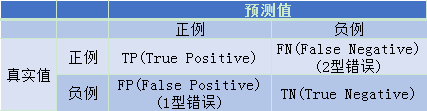True表示预测正确，False表示预测错误，将负例错误预测称为1型错误，将正例错误的预测成负例被称为2型错误。

• 准确率(Accuracy)=预测正确的样本数/总样本数

• 精确率(Precision)=TP/预测为正例的样本数

• 召回率(Recall)=TP/样本真实值为正例的样本数

• F值=Precision和Recall的调和平均值

F值是精确率和召回率的调和平均数。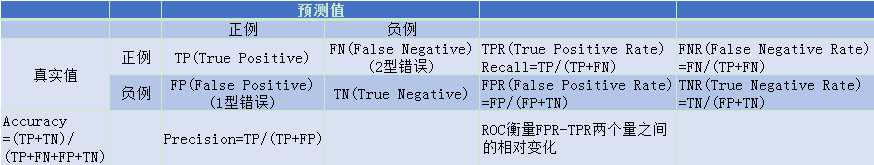## 混淆矩阵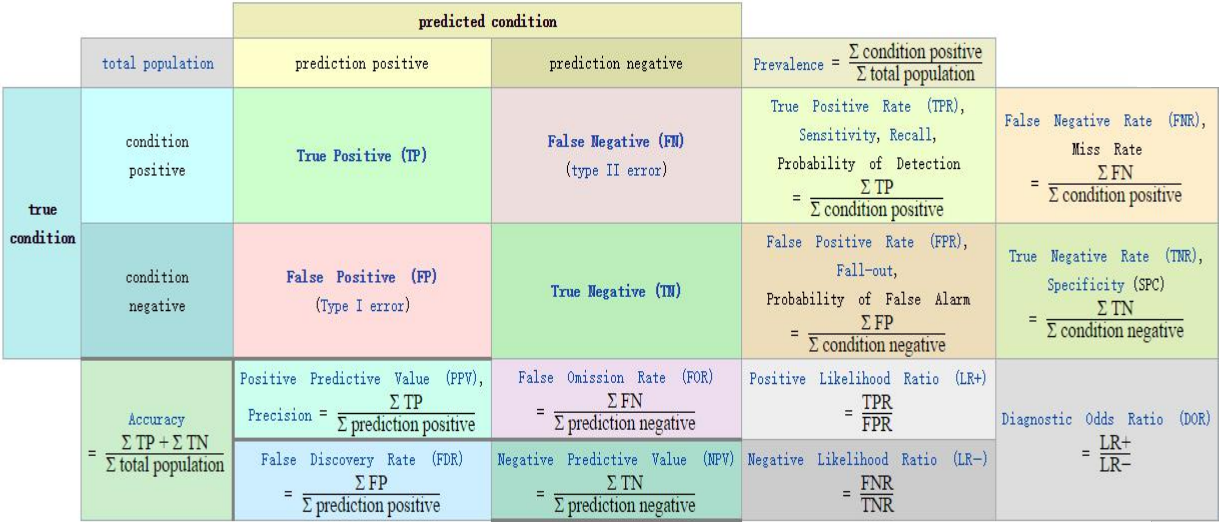## 准确率

Accuracy  = T P + T N T P + F P + F N + T N \text { Accuracy }=\frac{\mathrm{TP}+\mathrm{TN}}{\mathrm{TP}+\mathrm{FP}+\mathrm{FN}+\mathrm{TN}}

## 精确率和召回率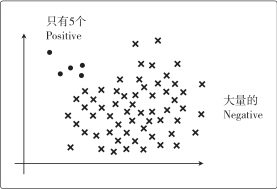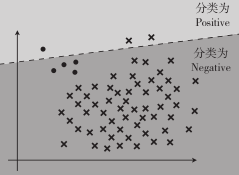Precision  = T P T P + F P \text { Precision }=\frac{\mathrm{TP}}{\mathrm{TP}+\mathrm{FP}}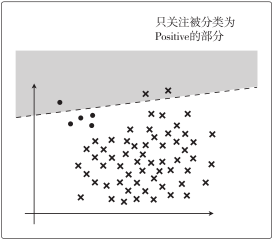Recall  = T P T P + F N \text { Recall }=\frac{\mathrm{TP}}{\mathrm{TP}+\mathrm{FN}}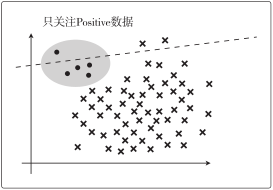## F 值

Fmeasure  = 2 1  Precision  + 1  Recall  \Huge \text { Fmeasure }=\frac{2}{\frac{1}{\text { Precision }}+\frac{1}{\text { Recall }}}

Freasure  = 2 ⋅  Precision  ⋅  Recall   Precision  +  Recall  \text { Freasure }=\frac{2 \cdot \text { Precision } \cdot \text { Recall }}{\text { Precision }+\text { Recall }}

WeightedFmeasure  = ( 1 + β 2 ) ⋅  Precision  ⋅  Recall  β 2 ⋅  Precision  +  Recall  \text { WeightedFmeasure }=\frac{\left(1+\beta^{2}\right) \cdot \text { Precision } \cdot \text { Recall }}{\beta^{2} \cdot \text { Precision }+\text { Recall }}

## ROC与AUC

ROC（Receiver Operating Characteristic） 描述的是FPR-TPR两个量之间的相对变化情况，纵轴是“真正率”（True Positive Rate 简称TPR），横轴是“假正率” （False Positive Rate 简称FPR）。ROC反映了TPR随着FPR递增的情况下，谁增长得更快，快多少的问题。TPR增长得越快，曲线越往上屈，AUC就越大，反映了模型的分类性能就越好。

AUC（Area Under Curve）被定义为ROC曲线下的面积，显然这个面积的数值不会大于1。又由于ROC曲线一般都处于y=x这条直线的上方，所以AUC的取值范围在0.5和1之间。AUC作为ROC曲线的具体数值可以直观的评价分类器的好坏，值越大越好。

• AUC = 1，是完美分类器，采用这个预测模型时，不管设定什么阈值都能得出完美预测。绝大多数预测的场合，不存在完美分类器。
• 0.5 < AUC < 1，优于随机猜测。这个分类器（模型）妥善设定阈值的话，能有预测价值。
• AUC = 0.5，跟随机猜测一样（例：丢铜板），模型没有预测价值。
• AUC < 0.5，比随机猜测还差；但只要总是反预测而行，就优于随机猜测。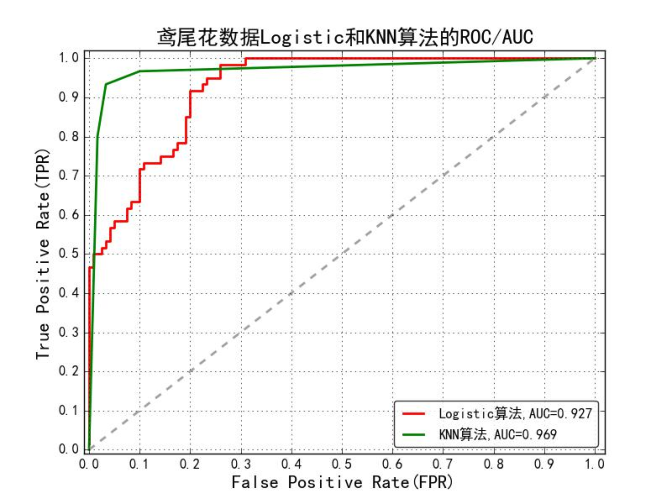## 各评价指标对于的sklearn函数

Precision精确度from sklearn.metrics import precision_score
Recall召回率from sklearn.metrics import recall_score
F1F1指标from sklearn.metrics import f1_score
Confusion Matrix混淆矩阵from sklearn.metrics import confusion_matrix
ROCROC曲线from sklearn.metrics import roc
AUCROC曲线下的面积from sklearn.metrics import auc